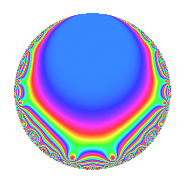# Properties

 Label 5610.2.a.caLevel 5610 Weight 2 Character orbit 5610.a Self dual Yes Analytic conductor 44.796 Analytic rank 0 Dimension 3 CM No Inner twists 1

# Related objects

## Newspace parameters

 Level: $$N$$ = $$5610 = 2 \cdot 3 \cdot 5 \cdot 11 \cdot 17$$ Weight: $$k$$ = $$2$$ Character orbit: $$[\chi]$$ = 5610.a (trivial)

## Newform invariants

 Self dual: Yes Analytic conductor: $$44.7960755339$$ Analytic rank: $$0$$ Dimension: $$3$$ Coefficient field: 3.3.961.1 Coefficient ring: $$\Z[a_1, \ldots, a_{7}]$$ Coefficient ring index: $$2$$ Fricke sign: $$-1$$ Sato-Tate group: $\mathrm{SU}(2)$

## $q$-expansion

Coefficients of the $$q$$-expansion are expressed in terms of a basis $$1,\beta_1,\beta_2$$ for the coefficient ring described below. We also show the integral $$q$$-expansion of the trace form.

 $$f(q)$$ $$=$$ $$q - q^{2} + q^{3} + q^{4} + q^{5} - q^{6} + \beta_{1} q^{7} - q^{8} + q^{9} +O(q^{10})$$ $$q - q^{2} + q^{3} + q^{4} + q^{5} - q^{6} + \beta_{1} q^{7} - q^{8} + q^{9} - q^{10} + q^{11} + q^{12} + ( -1 + \beta_{1} - \beta_{2} ) q^{13} -\beta_{1} q^{14} + q^{15} + q^{16} - q^{17} - q^{18} + ( 1 - \beta_{1} - \beta_{2} ) q^{19} + q^{20} + \beta_{1} q^{21} - q^{22} + ( 4 + \beta_{1} ) q^{23} - q^{24} + q^{25} + ( 1 - \beta_{1} + \beta_{2} ) q^{26} + q^{27} + \beta_{1} q^{28} + ( 1 + \beta_{2} ) q^{29} - q^{30} + ( 1 - \beta_{2} ) q^{31} - q^{32} + q^{33} + q^{34} + \beta_{1} q^{35} + q^{36} + 6 q^{37} + ( -1 + \beta_{1} + \beta_{2} ) q^{38} + ( -1 + \beta_{1} - \beta_{2} ) q^{39} - q^{40} + ( -2 - 2 \beta_{1} ) q^{41} -\beta_{1} q^{42} + \beta_{1} q^{43} + q^{44} + q^{45} + ( -4 - \beta_{1} ) q^{46} -4 q^{47} + q^{48} + \beta_{2} q^{49} - q^{50} - q^{51} + ( -1 + \beta_{1} - \beta_{2} ) q^{52} + ( 3 + \beta_{1} - \beta_{2} ) q^{53} - q^{54} + q^{55} -\beta_{1} q^{56} + ( 1 - \beta_{1} - \beta_{2} ) q^{57} + ( -1 - \beta_{2} ) q^{58} + ( -1 - \beta_{1} + \beta_{2} ) q^{59} + q^{60} + 2 q^{61} + ( -1 + \beta_{2} ) q^{62} + \beta_{1} q^{63} + q^{64} + ( -1 + \beta_{1} - \beta_{2} ) q^{65} - q^{66} + ( -2 + 2 \beta_{1} + 2 \beta_{2} ) q^{67} - q^{68} + ( 4 + \beta_{1} ) q^{69} -\beta_{1} q^{70} + ( 7 - \beta_{1} + \beta_{2} ) q^{71} - q^{72} + ( -3 + \beta_{1} + \beta_{2} ) q^{73} -6 q^{74} + q^{75} + ( 1 - \beta_{1} - \beta_{2} ) q^{76} + \beta_{1} q^{77} + ( 1 - \beta_{1} + \beta_{2} ) q^{78} + ( 3 + \beta_{1} + \beta_{2} ) q^{79} + q^{80} + q^{81} + ( 2 + 2 \beta_{1} ) q^{82} + ( 4 - 4 \beta_{1} ) q^{83} + \beta_{1} q^{84} - q^{85} -\beta_{1} q^{86} + ( 1 + \beta_{2} ) q^{87} - q^{88} + ( 2 + 2 \beta_{1} ) q^{89} - q^{90} + ( 8 - 4 \beta_{1} ) q^{91} + ( 4 + \beta_{1} ) q^{92} + ( 1 - \beta_{2} ) q^{93} + 4 q^{94} + ( 1 - \beta_{1} - \beta_{2} ) q^{95} - q^{96} + ( 1 + 2 \beta_{1} + \beta_{2} ) q^{97} -\beta_{2} q^{98} + q^{99} +O(q^{100})$$ $$\operatorname{Tr}(f)(q)$$ $$=$$ $$3q - 3q^{2} + 3q^{3} + 3q^{4} + 3q^{5} - 3q^{6} + q^{7} - 3q^{8} + 3q^{9} + O(q^{10})$$ $$3q - 3q^{2} + 3q^{3} + 3q^{4} + 3q^{5} - 3q^{6} + q^{7} - 3q^{8} + 3q^{9} - 3q^{10} + 3q^{11} + 3q^{12} - 2q^{13} - q^{14} + 3q^{15} + 3q^{16} - 3q^{17} - 3q^{18} + 2q^{19} + 3q^{20} + q^{21} - 3q^{22} + 13q^{23} - 3q^{24} + 3q^{25} + 2q^{26} + 3q^{27} + q^{28} + 3q^{29} - 3q^{30} + 3q^{31} - 3q^{32} + 3q^{33} + 3q^{34} + q^{35} + 3q^{36} + 18q^{37} - 2q^{38} - 2q^{39} - 3q^{40} - 8q^{41} - q^{42} + q^{43} + 3q^{44} + 3q^{45} - 13q^{46} - 12q^{47} + 3q^{48} - 3q^{50} - 3q^{51} - 2q^{52} + 10q^{53} - 3q^{54} + 3q^{55} - q^{56} + 2q^{57} - 3q^{58} - 4q^{59} + 3q^{60} + 6q^{61} - 3q^{62} + q^{63} + 3q^{64} - 2q^{65} - 3q^{66} - 4q^{67} - 3q^{68} + 13q^{69} - q^{70} + 20q^{71} - 3q^{72} - 8q^{73} - 18q^{74} + 3q^{75} + 2q^{76} + q^{77} + 2q^{78} + 10q^{79} + 3q^{80} + 3q^{81} + 8q^{82} + 8q^{83} + q^{84} - 3q^{85} - q^{86} + 3q^{87} - 3q^{88} + 8q^{89} - 3q^{90} + 20q^{91} + 13q^{92} + 3q^{93} + 12q^{94} + 2q^{95} - 3q^{96} + 5q^{97} + 3q^{99} + O(q^{100})$$

Basis of coefficient ring in terms of a root $$\nu$$ of $$x^{3} - x^{2} - 10 x + 8$$:

 $$\beta_{0}$$ $$=$$ $$1$$ $$\beta_{1}$$ $$=$$ $$\nu$$ $$\beta_{2}$$ $$=$$ $$\nu^{2} - 7$$
 $$1$$ $$=$$ $$\beta_0$$ $$\nu$$ $$=$$ $$\beta_{1}$$ $$\nu^{2}$$ $$=$$ $$\beta_{2} + 7$$

## Embeddings

For each embedding $$\iota_m$$ of the coefficient field, the values $$\iota_m(a_n)$$ are shown below.

For more information on an embedded modular form you can click on its label.

Label $$\iota_m(\nu)$$ $$a_{2}$$ $$a_{3}$$ $$a_{4}$$ $$a_{5}$$ $$a_{6}$$ $$a_{7}$$ $$a_{8}$$ $$a_{9}$$ $$a_{10}$$
1.1
 −3.08387 0.786802 3.29707
−1.00000 1.00000 1.00000 1.00000 −1.00000 −3.08387 −1.00000 1.00000 −1.00000
1.2 −1.00000 1.00000 1.00000 1.00000 −1.00000 0.786802 −1.00000 1.00000 −1.00000
1.3 −1.00000 1.00000 1.00000 1.00000 −1.00000 3.29707 −1.00000 1.00000 −1.00000
 $$n$$: e.g. 2-40 or 990-1000 Significant digits: Format: Complex embeddings Normalized embeddings Satake parameters Satake angles

## Inner twists

This newform does not admit any (nontrivial) inner twists.

## Atkin-Lehner signs

$$p$$ Sign
$$2$$ $$1$$
$$3$$ $$-1$$
$$5$$ $$-1$$
$$11$$ $$-1$$
$$17$$ $$1$$

## Hecke kernels

This newform can be constructed as the intersection of the kernels of the following linear operators acting on $$S_{2}^{\mathrm{new}}(\Gamma_0(5610))$$:

 $$T_{7}^{3} - T_{7}^{2} - 10 T_{7} + 8$$ $$T_{13}^{3} + 2 T_{13}^{2} - 40 T_{13} - 64$$ $$T_{19}^{3} - 2 T_{19}^{2} - 40 T_{19} + 64$$ $$T_{23}^{3} - 13 T_{23}^{2} + 46 T_{23} - 32$$